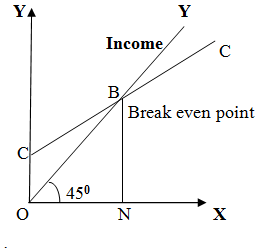### State and explain Keynesian 'Psychological Law of Consumption'

Meaning: -J.M KEYNES introduced the concept of consumption function is his book “The General Theory of employment, interest and money”. Keynes developed the ‘Psychological Law of Consumption”.

This law indicates.
·        When the income increases, the consumption expenditure also increases. But consumption expenditure increase at a lower rate as compared to increase in income.
·        The increase in income results in increase in consumption as savings. This is because; the rate of change in consumption is less than change in income.

The consumption function indicates the functional relationship between consumption and the level of disposable income. Keynes states that other things being constant (ceteris paribus) consumption is a function of income. This can be expressed as follows:
C = f (Y)

Where,         C = Consumption expenditure
f   = functional relationship
Y = income

The consumption function indicates that a change in consumption possible with a change in income. However, consumption need not always dependent on income. The consumption function, which shows the relationship between consumption and income, is explained in the diagram below.In the above diagram, ‘CC’ curve represents consumption curve of consumption function and ‘OY’ curve represents income, which drawn at 45O and it shows that at any point income is equal to consumption. It shows that if income is zero the consumption is not zero but it’s equal to ‘OC’. This ‘OC’ amount of consumption represents the autonomous part of consumption, which is independent of income. Even if income is zero, there is still consumption expenditure and it is done through borrowings. As the income increase and at ‘ON’ level of income, the consumption curve ‘CC’ Intersects the income curve ‘OY’ at point ‘B’ and it is called breakeven point.
At break even point, income = consumption below break even point, there is dis-saving, i.e., the consumption expenditure is more than income. Above break even point, there is saving, i.e income is more than consumption.

Consumption Schedule.

The consumption function is also called as propensity to consume. The propensity to consume is the natural tendency to consume. Consumption depends on level of income. When income increases, consumption also increase but at a lower rate.

Consumption function schedule (Rs. Crore)

 Income (Y) Consumption (C) 10000 15000 20000 25000 30000 13000 15000 17000 19000 21000

From the above schedule, it is clear that as the income increases, the consumption also increases, but at a lower rate. The schedule also indicates that there can be autonomous consumption. For instance, when income was Rs. 10000 crore, the consumption expenditure was Rs. 13000 crore, i.e., Rs. 3000 autonomous consumption, which may be done through borrowing or past savings.